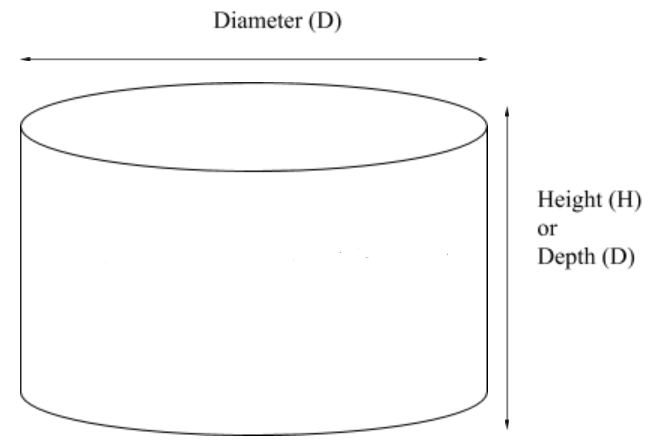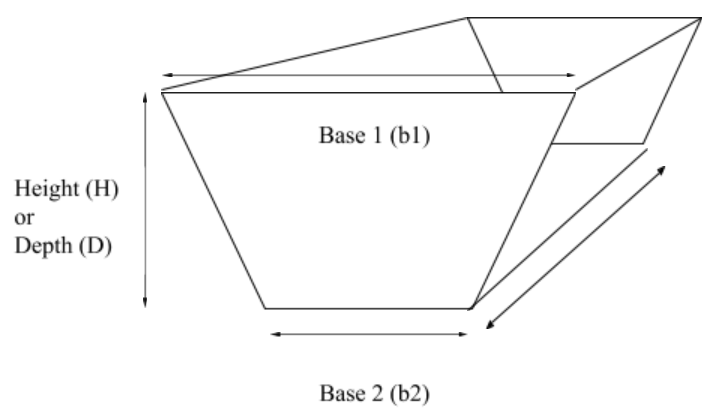# 6.2: Volumes

$$\newcommand{\vecs}{\overset { \rightharpoonup} {\mathbf{#1}} }$$ $$\newcommand{\vecd}{\overset{-\!-\!\rightharpoonup}{\vphantom{a}\smash {#1}}}$$$$\newcommand{\id}{\mathrm{id}}$$ $$\newcommand{\Span}{\mathrm{span}}$$ $$\newcommand{\kernel}{\mathrm{null}\,}$$ $$\newcommand{\range}{\mathrm{range}\,}$$ $$\newcommand{\RealPart}{\mathrm{Re}}$$ $$\newcommand{\ImaginaryPart}{\mathrm{Im}}$$ $$\newcommand{\Argument}{\mathrm{Arg}}$$ $$\newcommand{\norm}{\| #1 \|}$$ $$\newcommand{\inner}{\langle #1, #2 \rangle}$$ $$\newcommand{\Span}{\mathrm{span}}$$ $$\newcommand{\id}{\mathrm{id}}$$ $$\newcommand{\Span}{\mathrm{span}}$$ $$\newcommand{\kernel}{\mathrm{null}\,}$$ $$\newcommand{\range}{\mathrm{range}\,}$$ $$\newcommand{\RealPart}{\mathrm{Re}}$$ $$\newcommand{\ImaginaryPart}{\mathrm{Im}}$$ $$\newcommand{\Argument}{\mathrm{Arg}}$$ $$\newcommand{\norm}{\| #1 \|}$$ $$\newcommand{\inner}{\langle #1, #2 \rangle}$$ $$\newcommand{\Span}{\mathrm{span}}$$$$\newcommand{\AA}{\unicode[.8,0]{x212B}}$$

## Cylinder

Volume of a cylinder = $$0.785 \times D^{2} \times H$$Figure $$\PageIndex{1}$$: Volume of a cylinder = $$0.785 \times D^{2} \times H$$

The volume of a cylinder uses the area formula of a circle with the addition of a third dimension. Notice that a cylinder can have either a depth or a height. They are one and the same. It all depends on your perspective. In fact all 3 dimensional structures can have a depth or a height.

## Rectangle

Volume of a rectangle = $$L \times W \times H$$Figure $$\PageIndex{2}$$: Volume of a rectangle = $$L \times W \times H$$

## Trapezoid

Volume of a trapezoid = $$\dfrac{b1+b2}{2} \times H \times L$$Figure $$\PageIndex{3}$$: Volume of a trapezoid = $$\dfrac{b1+b2}{2} \times H \times L$$

There is something to keep in mind when calculating volumes. Most units in waterworks are given in “feet.” The length of pipe, the height of water tanks, the depths of aqueducts are all typically measured in feet. Therefore, calculating the volume for these geometric shapes result in cubic feet as the unit of measure. However, sometimes measurements are given in “inches” and will need to be converted to feet before solving a problem.

## Exercise 6.2

Solve the following

1. What is the area of a circle with a 10 foot diameter?
2. What is the area of a circle with a 10 inch diameter?
3. What is the area of a rectangle with a depth of 10 feet and a width of 20 feet?
4. What is the area of a trapezoid that has a distance across the base of 5 feet and a distance across the top of the water level of 7 feet. The water depth is 4 feet.
5. Calculate the volume of a pipe that has a 12” diameter and is 10 feet long.
6. How many gallons of water can fit in a storage tank that is 40 feet tall and has a 90 foot diameter? (Remember one cubic foot equals 7.48 gallons)
7. A rectangular basin is 100 feet long, 40 feet wide and has a depth of 25 feet. If the water level is 3 feet from the top, how much water is in the basin?
8. A one mile long water aqueduct is 10 feet deep and is half full. The width across the water level is 7 feet and the width at the base is 5 feet. How much water is in the aqueduct? (Remember that one mile equals 5,280 feet)
9. A 75 foot tall water tank is ¾ full and has a 30 foot diameter. How many gallons of water are in it?
10. What is the capacity of a water basin that is 200 feet long, 40 feet wide, and 30 feet deep?
11. A water tank is being emptied at a rate of 1 foot per hour. If the tank has a diameter of 100 feet, how many gallons will be emptied in 4 hours?
12. A reservoir with the following dimensions (75 feet long, 20 feet wide, 10 feet deep) is 35% empty. How many gallons of water are in the reservoir?

6.2: Volumes is shared under a CC BY license and was authored, remixed, and/or curated by LibreTexts.